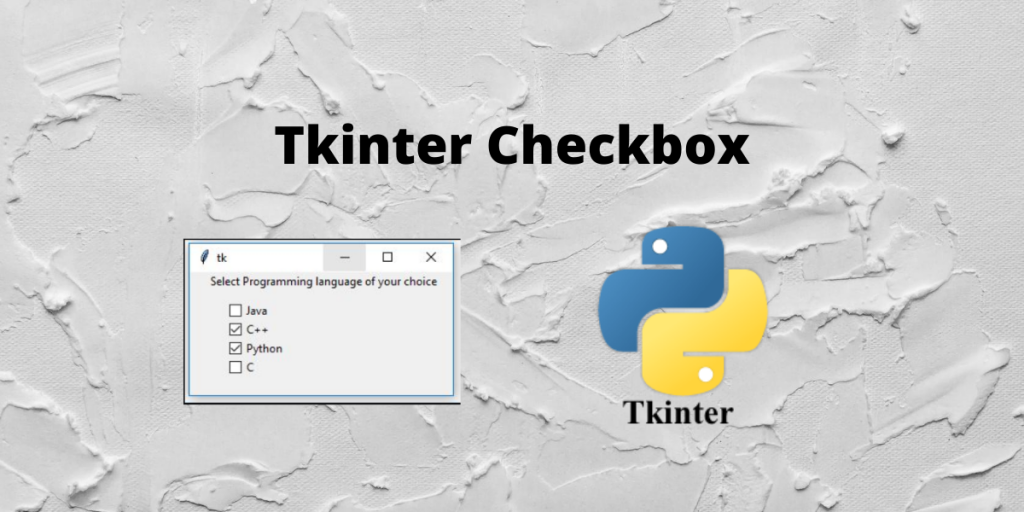# Tkinter Checkbox: An Easy ReferenceA tkinter checkbox can be created using the tkinter checkbutton widget. It allow users to select multiple options or choices from a number of different options. They are different from a radio button because in a radio button users can make only one choice, but checkbox allows multiple choices.

They are seen as square boxes containing white spaces. On the selection of a checkbox, a tick mark appears inside the box.

## Basic Implementation of Tkinter Checkbutton

Firstly, we will import the Tkinter module and initialize the geometry for the frame.

```from tkinter import *

root =Tk()

root.geometry("400x400+120+120")
root.mainloop()
```

Now that we have allotted the frame and hence we will place a checkbox using the Checkbox function. This is shown below. We will then use the pack() function to place this widget in the Tkinter frame.

```c = Checkbutton(root, text = "Python")
c.pack()

c1 = Checkbutton(root, text = "C++")
c1.pack()

c2 = Checkbutton(root, text = "C")
c2.pack()

```

The above code just displays three simple checkboxes with the text beside it. It contains no functionality because there is not a function being triggered.

Shown below is the entire code for the simple checkbox example. Do try it out and let us know your comments in the comment section at the end of this page.

```from tkinter import *

root =Tk()

c = Checkbutton(root, text = "Python")
c.pack()

c1 = Checkbutton(root, text = "C++")
c1.pack()

c2 = Checkbutton(root, text = "C")
c2.pack()

root.geometry("400x400+120+120")
root.mainloop()
```

The output of the above code is as shown below.

## Storing Tkinter Checkbox Value in a Variable

Firstly, we will import `tkinter` and initialize the root using the `Tk()` function. We also determine the size of the frame as well.

```from tkinter import *

root = Tk()

root.geometry("400x400+120+120")
root.mainloop()
```

We will place a checkbox and button on the frame and it is as shown below. We initialize a variable `i` and this `i` prints 0 if the checkbox is unchecked and 1 if the checkbox is checked on the console.

```root = Tk()
i = IntVar()

c = Checkbutton(root, text = "Python", variable=i)
c.pack()

b.pack()

```

The `click_me()` function that is triggered after the click after the button prints the `i` value stored in the variable for the checkbox.

```def click_me():
print(i.get())
```

The illustration of the whole code is as shown below. The screenshot of the output is also uploaded.

```from tkinter import *

def click_me():
print(i.get())

root =Tk()
i=IntVar()
c = Checkbutton(root, text = "Python", variable=i)
c.pack()

b.pack()

root.geometry("400x400+120+120")
root.mainloop()
```

The output of the above code is as shown below.